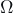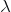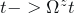# Solid state physics could teach us how to quantize gravity: Horava’s theory

One of hottest topics in theoretical physics today is, of course, quantum gravity. The fact that, almost a hundred years after the born of the quantum theory, we still not have a functional theory that describes gravity at a microscopic level has become one of the most attractive problems for the majority of physicists (even I am dedicating my Ph. D to explore this problem). During the last forty years, a huge amount of alternatives to the naive quantization of General Relativity, which we will see in a moment that it is not a valid option, have appeared and lead to some of the most interesting theories born at the twentieth century, being string theory and the holographic principle some well known examples.So, what is the problem with General Relativity (GR) and why we cannot quantize it in the same way as the other interactions we know? The main issue is that, apart from some interpretation details that I do not know if they are real problems, GR is non-renormalizable. When we quantize a theory, every physical quantity can be regarded as a Taylor expansion where the zeroth order is the classical prediction for that quantity and any other order is known to be a quantum correction. These quantum corrections can be regarded as modifications of the classical theory, in the sense that the quantum dynamics impose the equations of movement (or the lagrangian if we want to be more precise) to be different from their classical expressions. Thus, a quantum theory is only well-defined if we are able to compute such corrections; and here the main issue with GR arises. As opposite to the other forces of nature we know, GR has an infinite number of contributions to every order correction, making its computation impossible and producing a divergent theory (every physical magnitude is predicted to be infinite) at the last.

In order to address this problem, a thousand of alternatives have been studied, from the idea of changing the quantization procedure (Loop Quantum Gravity, but with no real achievements) to the proposal of every particle to be actually a string (String Theory, which is a great theoretical framework), passing through a lot of different field theory alternatives such as Dilaton Gravity, Bigravity, Unimodular Gravity, Massive Gravity, F(R) Gravity and so on. Of this latter type is the theory which Petr Horava 1 proposed back in 2009 and which is known as Horava-Lifshitz Gravity.

If I had chosen Horava’s theory as the topic of this post is because it has two main characteristics that attract me a lot. First, Horava’s idea is inspired by the field most of Quantum Field Theory ideas have come from, Solid State Physics; and by the concept of Critical Lifshitz points (that is where the Lifshitz surname of the theory comes from); and secondly, because it can be reduced to a change of the symmetries of nature, in the same sense as Special Relativity can be regarded as a change of Galileo’s symmetry by Lorentz one or General Relativity can be understood as the inclusion of diffeomorphisms as a new symmetry of the spacetime.

What Horava proposes is to substitute Lorentz (or diffeomorphism if we refer to the general case) invariance by a new symmetry performed in such as way that if we rescale all lengths by a factorand the time byto the z, the theory will be invariant. Such anisotropic scaling symmetry has the fancy property to introduce a new parameterin the kinetic energy term of the gravitational field and it is this parameter where all the magic relays on. The trick is that it is a dynamical quantity of the theory and therefore it is susceptible of receiving quantum corrections to its classical expectation value. Horava’s idea is then to choose the terms in the potential energy to make the value of this lambda parameter flow to one in the low energy limit, which is the value that collapses the theory to General Relativity and reduces the new symmetry to a democraticrescaling to both time and space, while maintaining the full symmetry () at high energies, protecting the infinites of usual GR to appear and defining a full renormalizable theory of quantum gravity. It sounds so good to be true, really? And it is.

The first problem of this proposal is that the number of terms one can include in the expression for the potential energy of the theory is enormous. Horava proposed a simple solution to this, called detailed balance prescription, which reduces the number of terms to only two and it is motivated as well as the full theory by some solid state physics ideas. However, soon after Horava’s original paper was released, D. Blas et al. showed that this choice had a big problem related to the degrees of freedom propagated by the theory.

In general, a theory of quantum gravity propagates not only a spin 2 particle but only a spin 0 one (a Higgs boson-like particle). General Relativity is the simplest theory and propagates only the spin 2 particle (the graviton) but other theories include both bosons. The problem with Horava’s proposal found by Blas and his fellows 2 is that the spin 0 particle becomes strongly coupled in the low energy limit, implying both that the matter content is different to the one observed by low energy experiments and also that the quantum corrections to the physical quantities related to this particle are infinite, so this do not get rid of the original problems of the naive quantization of GR and forces ourselves to not to use the detailed balance prescription in order to choose the potential terms.

This obviously makes Horava’s proposal of using detailed balance not useful at all but it does not completely discard the theory since there is still a big freedom to choose the potential terms and maybe a good election could solve any problem. However, not a solid proposal has appeared yet. Nevertheless, there is still hope since many theorists are actively working on it and future may depare some surprises. And even if Horava-Lifshitz results not to be the real theory of quantum gravity, it is still very interesting by itself and by its applications in the field of holography, in which it can be used to describe gravity duals of non relativistic theories.

Either way, the next years will be very interesting.

## References

1. P. Horava, “Quantum Gravity at a Lifshitz Point,”Phys. Rev. D 79 (2009) 084008 [arXiv:0901.3775 [hep-th]].
2. D.Blas, O.Pujolas and S.Sibiryakov, “On the Extra Mode and Inconsistency of Horava Gravity,” JHEP 0910 (2009) 029 [arXiv:0906.3046 [hep-th]].